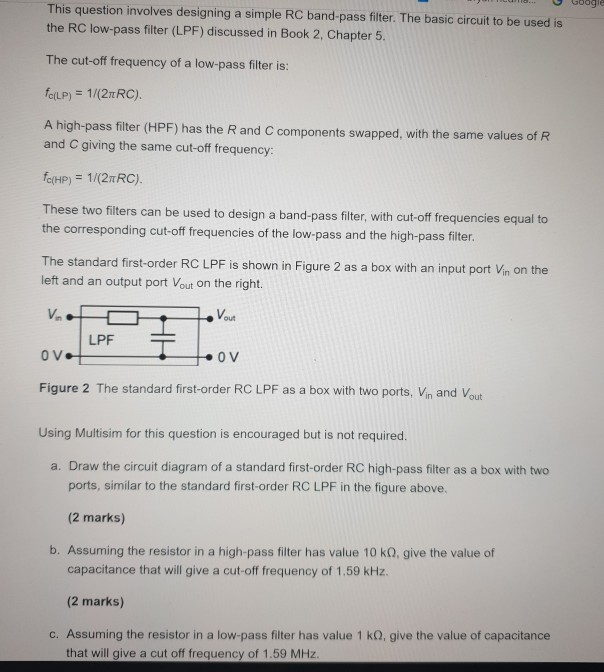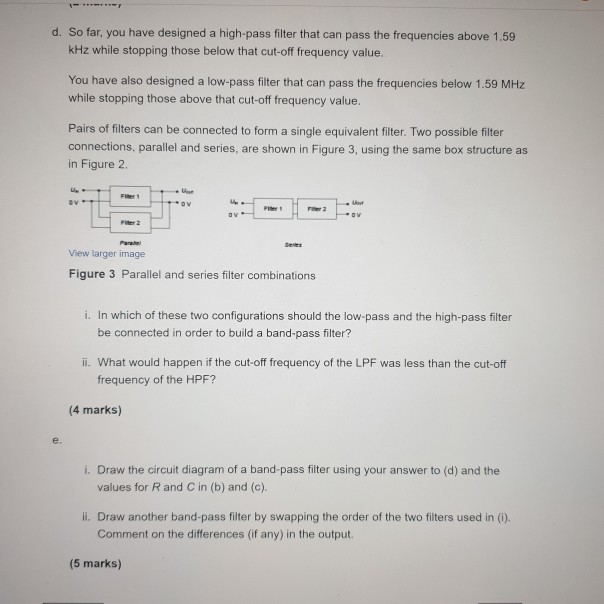# (Solved): ... Google This Question Involves Designing A Simple RC Band-pass Filter. The Basic Circuit To Be Us...... Google This question involves designing a simple RC band-pass filter. The basic circuit to be used is the RC low-pass filter (LPF) discussed in Book 2, Chapter 5. The cut-off frequency of a low-pass filter is: forLP) = 1/(2TRC). A high-pass filter (HPF) has the R and C components swapped, with the same values of R and C giving the same cut-off frequency: fc(HP) = 1/(2nRC). These two filters can be used to design a band-pass filter, with cut-off frequencies equal to the corresponding cut-off frequencies of the low-pass and the high-pass filter. The standard first-order RC LPF is shown in Figure 2 as a box with an input port Vin on the left and an output port Vout on the right. Vio -Von LPF OV OV Figure 2 The standard first-order RC LPF as a box with two ports, Vin and out Using Multisim for this question is encouraged but is not required. a. Draw the circuit diagram of a standard first-order RC high-pass filter as a box with two ports, similar to the standard first-order RC LPF in the figure above, (2 marks) b. Assuming the resistor in a high-pass filter has value 10 ko, give the value of capacitance that will give a cut-off frequency of 1.59 kHz. (2 marks) c. Assuming the resistor in a low-pass filter has value 1 kg, give the value of capacitance that will give a cut off frequency of 1.59 MHz. d. So far, you have designed a high-pass filter that can pass the frequencies above 1.59 kHz while stopping those below that cut-off frequency value. You have also designed a low-pass filter that can pass the frequencies below 1.59 MHz while stopping those above that cut-off frequency value. Pairs of filters can be connected to form a single equivalent filter. Two possible filter connections, parallel and series, are shown in Figure 3, using the same box structure as in Figure 2 OV View larger image Figure 3 Parallel and series filter combinations i. In which of these two configurations should the low-pass and the high-pass filter be connected in order to build a band-pass filter? ii. What would happen if the cut-off frequency of the LPF was less than the cut-off frequency of the HPF? (4 marks) i. Draw the circuit diagram of a band-pass filter using your answer to (d) and the values for R and C in (b) and (c). il Draw another band-pass filter by swapping the order of the two filters used in 0. Comment on the differences (if any) in the output. (5 marks)

We have an Answer from Expert